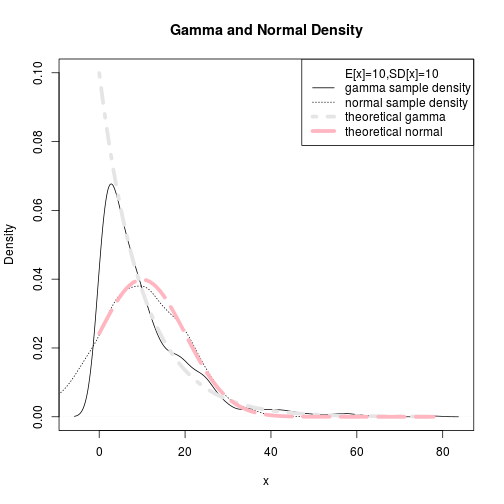``````## Title: distributions-GammaVersusNormal-1.R
## Author: Paul Johnson <pauljohn at ku.edu>
## Date posted: 2013-02-08
## Description. Explore the Gamma PDF and its comparison
## to the Normal distribution. This example shows some
## nice uses of lines and legends in the plot at the end.

## Change to TRUE if you want to write the file
SAVEME <- FALSE

pdf.options(family = "Times", paper = "special", onefile = TRUE)

## Ask for a big sheet of paper to draw on:
if (SAVEME) pdf(file = "gammaVnormal-1.1.pdf", width = 8.5, height = 11)
## If you are running interactively, I suggest you make the
## window larger.

## Partition display into 5 rows, 2 columns
par(mfcol=(c(5,2)))

#first, just leave the gamma "shape" equal to 1.  Then the
# mean = scale and the variance= scale*scale
for (i in 1:10) {
sc <- 0.5 + (i-1)
title <- paste("Histogram, shape=1, scale=", sc)
x <- rgamma(1250, shape = 1, scale = sc)
hist(x, breaks = 60, main = title)
}
````````````if (SAVEME) dev.off()

#what if we fix the scale=5 and shape varies?

if (SAVEME) pdf(file = "gammaVnormal-1.2.pdf", width = 8.5, height = 11)
par(mfcol=(c(5,2)))
for (i in 1:10) {
sc <- 5
sh = 0.5 + (i-1)
title <- paste("Histogram, shape=,",sh,"scale=5")
x <- rgamma(1250, shape = sh, scale = sc)
hist(x, breaks = 60, main = title)
}
````````````if (SAVEME) dev.off()

### Create a gamma and normal sample with same mean/SD
if (SAVEME) pdf(file = "gammaVnormal-1.3.pdf", width = 7, height = 7)
par(mfcol=c(1,1))
y <- rgamma(1000, scale = 10, shape = 1);
x <- rnorm(1000, mean = 10, sd = 10)
plot(x, y, main = "y a gamma,x a normal")
````````````if (SAVEME) dev.off()

### here's a fun exercise. Compare the observed density
if (SAVEME) pdf(file = "gammaVnormal-1.4.pdf", width = 8.5, height = 11)
plot(density(y), ylim=c(0,.1), lty = 2, xlab = "x", main = "Gamma and Normal Density")
dennorm <- density(x)
lines(dennorm, lty = 3)
legend("topright", legend=c("E[x]=10,SD[x]=10",
"gamma sample density",
"normal sample density"), lty = c(NA,2,3))
````````````if (SAVEME) dev.off()

### Now, start over, add 2 "true" density lines
if (SAVEME) pdf(file = "gammaVnormal-1.5.pdf", width = 7, height = 7)

plot(density(y), ylim = c(0,.1), lty = 1, xlab = "x",
main = "Gamma and Normal Density")

dennorm <- density(x)
lines(dennorm, lty = 3)

xrange <- seq(range(y), range(y), length.out = 100)

truedengamma <- dgamma(xrange, scale = 10, shape = 1)
lines(xrange, truedengamma, lty = 4,col = "gray90", lwd = 5)

truedennorm <- dnorm(xrange, mean = 10, sd = 10)
lines(xrange, truedennorm, lty = 5, col = "lightpink", lwd = 5)

legend("topright", legend = c("E[x]=10,SD[x]=10",
"gamma sample density", "normal sample density", "theoretical gamma",
"theoretical normal"), lty = c(NA,1,3,4,5), col =
c(1,1,1,"gray90","lightpink"), lwd = c(NA,1,1,5,5))
````````````if (SAVEME) dev.off()
``````